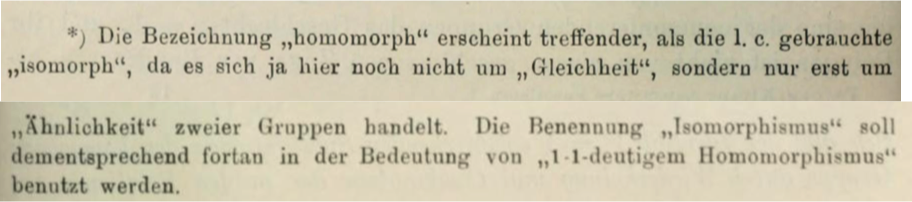# Whence “homomorphism” and “homomorphic”?

Today homomorphism (resp. isomorphism) means what Jordan (1870) had called isomorphism (resp. holoedric isomorphism). How did the switch happen?

“Homomorphic” (and “homomorphism” as “property of being homomorphic”) are e.g. in de Séguier (1904, pp. 65–66) and the last edition of Weber (1912, p. 195). “Homomorphism” as map of groups is e.g. in Schur (1924, p. 192). But none of these sound like a first.

I asked this on hsm a week ago, but got no answer there.

• Remark: the earliest uses site says 1935, but even in English this is predated by Pontrjagin (1934, p. 362), Tucker (1933, p. 196), and for algebra homomorphisms, Levitzki (1932, p. 382). – Francois Ziegler Sep 3 '17 at 21:46
• People were still using isomorphism onto and isomorphism into for surjective resp. injective homomorphisms, for far too long (IMHO). I've always found this weird, but I guess they were just not up to date with the change terminology. – David Roberts Sep 3 '17 at 21:52
• @DavidRoberts Indeed, e.g., van der Waerden’s Moderne Algebra (1930, p. 32) still laments the absence of stable terminology. – Francois Ziegler Sep 3 '17 at 22:11
• Note that in the linked paper by Jordan, "group" means "permutation group", i.e., something which more or less means a set endowed with a subgroup of its group of permutations. Isomorphic means isomorphic as groups (not as permutation group). In particular when he says "Problem: determine all groups isomorphic to a given group $G$", it more or less means "classify all (faithful) actions of $G$ [the underlying group, in the modern sense]". – YCor Sep 3 '17 at 22:14The term "homomorphic" seems more appropriate than the previously$^\ast$ used "isomorphic", because it refers not to "equality" but to "similarity" of two groups. The term "isomorphism" will therefore from now on be used in the sense of "1-to-1 homomorphism".
$^*$l.c. = loco citato — this seems to refer to Fricke and Klein's Vorlesungen über die Theorie der elliptischen Modulfunctionen (1890).